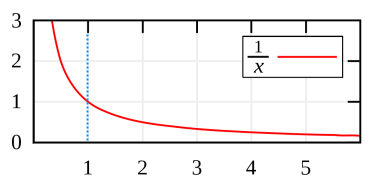Friday, January 20, 2012

The Impossible Vuvuzela

Mathematicians can describe a very strange theoretical object, which has two very paradoxic properties.

It looks like a horn, or maybe a trumpet. Hmmm, I guess it resembles one of those dreaded vuvuzelas most. It becomes thinner and thinner along its length — which, by the way, is infinite. You know what? Here's a picture:On the one hand this vuvuzela has an infinite surface area. Because as the vuvuzela becomes longer, its exterior and interior become bigger, thus this vuvuzela of infinite length has an infinite surface.

On the other hand, its volume approaches π. That's right, a finite value. Surprisingly, this vuvuzela of infinite length has an exact finite volume.If this vuvuzela would exist for real, it would be very strange indeed. You could fill it up with paint in its entirely and only need slightly over three buckets. But if you wanted to paint the interior, no matter how many buckets you'd buy it would never be enough. An endless supply of paint is needed to accomplish that... yet the paint you poured in should already be touching the interior!

Actually, who knows how that would work. Maybe the act of pouring in those few buckets of paint manages to cover an infinite surface area? In any case, it's paradoxical for sure!

A side note for the real world is that paint cannot be divided forever, so at some point the vuvuzela has become too narrow for the paint molecules to pass through. But the hard math doesn't have to worry about such trivial things as physics. This is purely theoretical, baby.

Details: It's easy to show how this figure is formed, so here we go.
1. Take the graph of y = 1 ÷ x.
That simply means that a position on the vertical y-axis is 1 divided with the position on the horizontal x-axis.The x-axis starts off from 1. Going onwards, the line quickly approaches 0 on the y-axis but never quite reaches it. It goes on like that forever.
2. Now to create the vuvuzela, the entire graph needs to be swung around its x-axis (one full revolution). The resulting 3D object looks like the first image in this post.
“One” can now use calculus and integrals to calculate the vuvuzela's surface area ‘A’ and volume ‘V’, based on its length ‘L’. For the record then, the limits are as such:
• A = (2 × π × the natural logarithm of L) → ∞   as L → ∞
• V = (π × (1 − 1÷L)) → π   as L → ∞